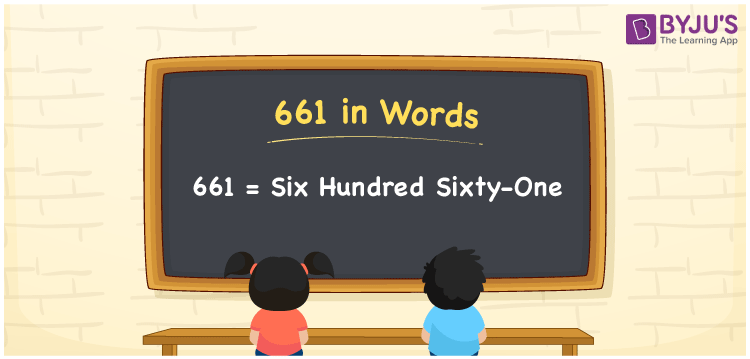# 661 in Words

661 in words is written as Six hundred sixty-one. In both the International System of Numerals and the Indian System of Numerals, 661 is written as Six hundred sixty-one. The number 661 is a Cardinal Number as it represents some quantity. For example, “there are 661 seats available”.

 661 in Words Six hundred sixty-one Six hundred sixty-one in Number 661

## 661 in English Words

We write 661 in English Words using the letters of the English alphabet. Therefore, we read 661 in English as “Six hundred sixty-one.”## How to Write 661 in Words?

To write 661 in words, we shall use the place value chart. In the place value chart, write 6 in the hundreds and tens, and 1 in the ones, respectively. Now let us make a place value chart to write the number 661 in words.

 Hundreds Tens Ones 6 6 1

Thus, we can write the expanded form as

6 × Hundred + 6 × Ten + 1 × One

= 6 × 100 + 6 × 10 + 1 × 1

= 600 + 60 + 1

= 661

= Six hundred sixty-one.

661 is a natural number, the successor of 660 and the predecessor of 662.

661 in words – Six hundred sixty-one

• Is 661 an odd number? – Yes
• Is 661 an even number? – No
• Is 661 a perfect square number? – No
• Is 661 a perfect cube number? – No
• Is 661 a prime number? – Yes
• Is 661 a composite number? – No

## Frequently Asked Questions on 661 in Words

Q1

### How to write 661 in words?

661 in words is written as Six hundred sixty-one.
Q2

### How to write 661 in the International and Indian System of Numerals?

In both, the system of numerals, 661 in words, is written as Six hundred sixty-one.
Q3

### What is the preceding number of 661?

The number that precedes 661 is 660.